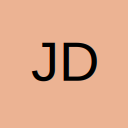large combination from small set

• Hello all, I was hoping it might be possible to look at a set of letters (atcg) and list all possible combinations to n
eg if n = 5

aaaaa
taaaa
caaaa
gaaaa
ataaa
ttaaa

etc... up to ggggg

the application is to look at possible combinations of nucleid acids, I will then use functions to calculate exact mass from the combinations and use it as a lookup database. I still have not worked out if the number of combinations will be too large at n = 20

I have seen a few examples of combinations where n is less than the number of items in the set but cannot find any way of doing this.

Thankyou for any help

Justin

• Re: large combination from small set

You can use a recursive procedure, soemthing like:

[vba]
Sub Wrapper()

Dim strLetters As String
Dim lngN As Long

strLetters = "atcg"

lngN = 5

Inner lngN, strLetters

End Sub

Sub Inner(ByVal lngN As Long, ByVal strLetters As String, Optional ByVal strAnswer As String = "")

Dim lngLoop As Long
Dim strTemp As String

If lngN = 0 Then
Debug.Print strAnswer 'or whatever you want to do with it
Else
For lngLoop = 1 To Len(strLetters)
strTemp = strAnswer & Mid(strLetters, lngLoop, 1)
Inner lngN - 1, strLetters, strTemp
Next
End If

End Sub
[/vba]

HTH

TJ

• Re: large combination from small set

Hi Tinyjack,

Thankyou for the reply... I cannot however get it to work. I copied the code to a module and ran it from a button. no errors but also nothing happened. How do I get the results to list down in the spread sheet?

Thanks again.

Justin

Also if you are a mathematician type, is there a way of doing it using formula.

There would be four constants A,T,C,G (the masses of the nucleotides)
four variables (WXYZ) which add up to the number of nucleotides (so if 20 W+X+Y+Z=20)

if M=total mass of the nucleotide string then

AW+XT+YC+ZG=M

would there be a way, if M is known, of calculating the possible values of WXY and Z.

hell I dont know.

• Re: large combination from small set

To see the results from the code you need to go into the VBE and look at the immediate window, which is where Debug.Print outputs to.

With regards to the second part of your post, try puting the following code into an empty workbook and then running Main(). This is just some code I knocked up and as such I am sure there is a lot that could be done to improve it's efficency.

ps Balls = nucleotides and Cups = four constants

[vba]
Option Explicit

Dim lngRow As Long

Sub Main()

Dim lngCups As Long
Dim lngBalls As Long

Application.ScreenUpdating = False

Sheet1.Rows("1:60000").Delete

lngBalls = 20
lngCups = 4

lngRow = 1

If doit(lngBalls, lngCups) < 60000 Then
Details lngBalls, lngCups
End If

Application.ScreenUpdating = True

End Sub

Public Function doit(ByVal lngBalls As Long, ByVal lngCups As Long) As Long

Dim lngloop As Long

If lngCups = 1 Or lngBalls = 0 Then
doit = 1
Else
For lngloop = 0 To lngBalls
doit = doit + doit(lngloop, lngCups - 1)
Next
End If

End Function

Sub Details(ByVal lngBalls As Long, ByVal lngCups As Long, Optional ByVal strAnswer As String = "")

Dim strTemp As String
Dim lngloop As Long

If lngCups = 1 Then
strTemp = strAnswer & ";" & CStr(lngBalls)
Else
strTemp = CStr(lngBalls)
End If
ElseIf lngBalls = 0 Then
For lngloop = 1 To lngCups
If Len(strTemp) > 0 Then
strTemp = strTemp & ";0"
Else
strTemp = "0"
End If
Next
Else
For lngloop = 0 To lngBalls
strTemp = strAnswer & ";" & CStr(lngBalls - lngloop)
Else
strTemp = CStr(lngBalls - lngloop)
End If
Details lngloop, lngCups - 1, strTemp
Next
End If

End Sub

Dim lngloop As Long

For lngloop = 0 To UBound(varAnswers)
Sheet1.Cells(lngRow, lngloop + 1).Value = varAnswers(lngloop)
Next

lngRow = lngRow + 1

End Sub
[/vba]

HTH

TJ